• 五个excel常用函数
2020-11-18 11:25:46

这次给大家带来几个Excel中的常用函数，在日常使用excel时，熟练掌握函数的运用技巧，使工作效率显著提高。

隔行添加颜色

将横向单元格每间隔1行添加颜色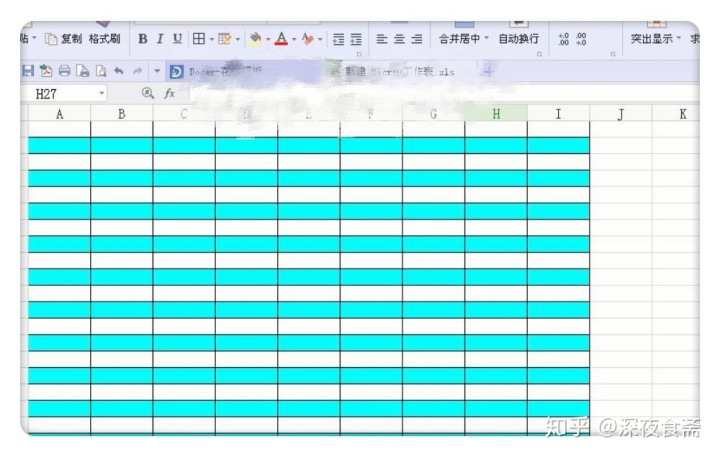点击（开始）（样式）--（条件格式）--（新建规则）--点击第六个选项--输入对应函数：=MOD(ROW(),2)=1--再点击（格式）--（填充）--添加（背景颜色）

计算数据排名

依次将单元格里面的数据从高到下，显示出各数据的排名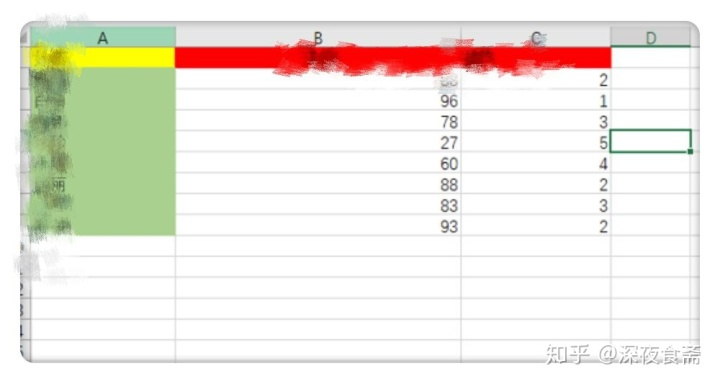在排名单元格中输入

函数:=SUMPRODUCT((B$2:B$7>B2)/COUNTIF(B$2:B$7,B$2:B$7))+1

将函数复制到对应的第一个单元格里面，然后再往下拉即可！

合并单元格内容

将单元格里面的内容全都合并在一个单元格里面

可以使用 CONCATENATE 函数CONCATENATE(文本1，文本2……)

文本1，文本2……是需要连接的字符文本或引用的单元格。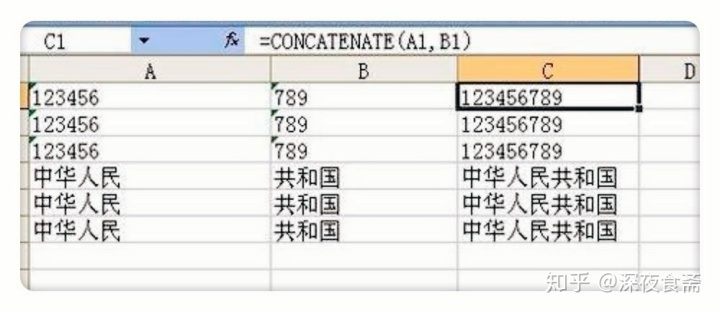假设合并A1 B1

可以输入函数=CONCATE5NATE(A1，B1)，然后将函数复制到对应的单元格里即可。

查找重复内容

我们可以使用Countif函数来一一统计每条数在所有数据中的个数，如果得出的个数大于1，则证明是重复数据，否则就是唯一数据。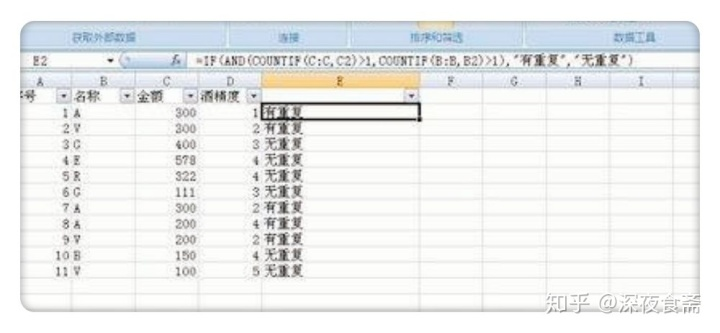然后用IF函数

输入公式=IF(COUNTIF(C:C,C2)>1,"重复","")。

提取不重复值(按上图）

在目标单元格中输入公式：=INDEX(E:E,2+MATCH(,COUNTIF(F$2:F2,E$3:E$9),))&""。 常用汇总函数 在对应的目标单元格中输入公式 基础汇总公式 （A假设） A列求和：=SUM(A:A) A列最小值：=MIN(A:A) A列最大值：=MAX (A:A) A列平均值：=AVERAGE(A:A) A列数值个数：=COUNT(A:A) 更多相关内容 • 主要适用于EXCEL入门者，快速了解EXCEL常用函数 • EXCEL常用函数公式大全与举例-举列常用函数.doc • Excel函数词典（483个函数说明） BETADIST，BETAINV，BINOMDIST • 今天和大家分享一组常用函数公式的使用方法，用心掌握这些函数，工作效率会大大提升。欢迎转发给更多有需要的人。一、相关概念(一)函数语法由函数名+括号+参数组成例： 求和函数：SUM(A1,B2,…) 。参数与参数之 间用... 今天和大家分享一组常用函数公式的使用方法，用心掌握这些函数，工作效率会大大提升。欢迎转发给更多有需要的人。 一、相关概念 (一)函数语法 由函数名+括号+参数组成 例： 求和函数：SUM(A1,B2,…) 。参数与参数之 间用逗号“,”隔开 (二)运算符 1. 公式运算符：加(+)、减(-)、乘(*)、除(/)、百分号(%)、乘幂(＾) 2. 比较运算符：大与(>)、小于(=) 、不等于(<>) 3. 引用运算符：区域运算符(：)、联合运算符(,) (三)单元格的相对引用与绝对引用 例： A1$A1 锁定第A列

A$1 锁定第1行$A$1 锁定第A列与第1行 二、常用函数 (一)数学函数 1. 求和 =SUM(数值1,数值2,……) 2. 条件求和 =SUMIF(查找的范围,条件(即对象),要求和的范围) 例：(1)=SUMIF(A1:A4,”>=200”,B1:B4) 函数意思：对第A1栏至A4栏中，大于等于200的数值对应的第B1列至B4列中数值求和 (2)=SUMIF(A1:A4,”<300”,C1:C4) 函数意思：对第A1栏至A4栏中，小于300的数值对应的第C1栏至C4栏中数值求和 3. 求个数 =COUNT(数值1,数值2,……) 例：(1) =COUNT(A1:A4) 函数意思：第A1栏至A4栏求个数 (2) =COUNT(A1:C4) 函数意思：第A1栏至C4栏求个数 4. 条件求个数 =COUNTIF(范围,条件) 例：(1) =COUNTIF(A1:A4，”<>200”) 函数意思：第A1栏至A4栏中不等于200的栏求个数 (2) =COUNTIF(A1:C4,”>=1000”) 函数意思：第A1栏至C4栏中大于等1000的栏求个数 5. 求算术平均数 =AVERAGE(数值1,数值2,……) 例：(1) =AVERAGE(A1,B2) (2) =AVERAGE(A1:A4) 6. 四舍五入函数 =ROUND(数值,保留的小数位数) 7. 排位函数 =RANK(数值,范围,序别) 1-升序 0-降序 例：(1) =RANK(A1,A1:A4,1) 函数意思：第A1栏在A1栏至A4栏中按升序排序，返回排名值。 (2) =RANK(A1,A1:A4,0) 函数意思：第A1栏在A1栏至A4栏中按降序排序，返回排名值。 8. 乘积函数 =PRODUCT(数值1,数值2,……) 9. 取绝对值 =ABS(数字) 10. 取整 =INT(数字) (二)逻辑函数 条件函数：=IF(条件,执行条件,执行条件假)—可执行七层嵌套 例： A1=5 A2=3 A3=6 A4=7 =IF(A1>A2,1,0)=1(真) =IF(A1 =IF(A1>A2,IF(A3>A4,8,9),1)=9 (三)文本函数 1. 截取函数： =LEFT(文本,截取长度)—从左边截取 =RIGHT(文本,截取长度)—从右边截取 =MID(文本,开始位,截取长度)—从中间截取 2. 计算字符长度：LEN(文本)—文本的长度 3. 合并字符函数：CONCATENATE(文本1,文本2,…) 4. 在字符串中查找特定字符：FIND(文本,范围,数值)—数值表示查找第几个 =FIND(“a”,“abcadeafga”,2)=4 =FIND(“a”,“abcadeafga”,3)=7 =FIND(“a”,“abcadeafga”,4)=10 5. 比较两个字符是否完全相符：EXACT(文本1,文本2) =EXACT(“a”,“a”)=TRUE =EXACT(“a”,“ab”)=FALSE =EXACT(“a”,“A”)=FALSE 6. 将数值转化为文本：TEXT(数值,参数) )—参数一般为0 7. 将数值型字符转换为数值：VALUE(数值文本) (四)判断公式 1. 把公式产生的错误显示为空 公式：C2=IFERROR(A2/B2,””) 说明：如果是错误值则显示为空，否则正常显示。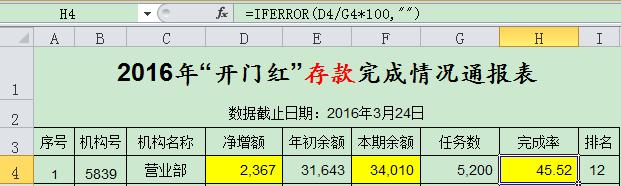2. IF多条件判断返回值 公式：C2=IF(AND(A2<500,B2=”未到期”),”补款”,””) 说明：两个条件同时成立用AND，任一个成立用OR函数。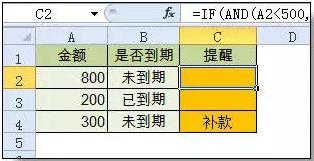(五)统计公式 1. 统计两个表格重复的内容 公式：B2=COUNTIF(Sheet15!A:A,A2) 说明：如果返回值大于0说明在另一个表中存在，0则不存在。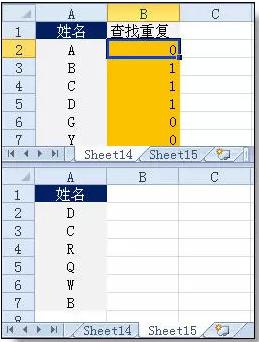2. 统计不重复的总人数 公式：C2=SUMPRODUCT(1/COUNTIF(A2:A8,A2:A8)) 说明：用COUNTIF统计出每人的出现次数，用1除的方式把出现次数变成分母，然后相加。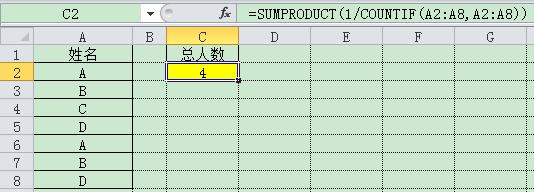(六)求和公式 1. 隔列求和 公式：H3=SUMIF($A$2:$G$2,H$2,A3:G3)

或者： =SUMPRODUCT((MOD(COLUMN(B3:G3),2)=0)*B3:G3)

说明：如果标题行没有规则用第2个公式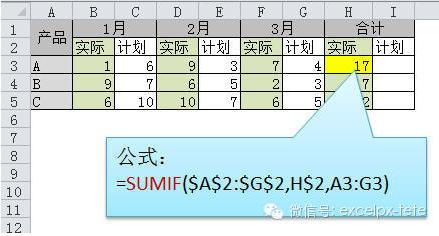2. 单条件求和

3. 单条件模糊求和

4. 多条件模糊求和

5. 多表相同位置求和

公式：B2=SUM(Sheet1:Sheet19!B2)

说明：在表中间删除或添加表后，公式结果会自动更新。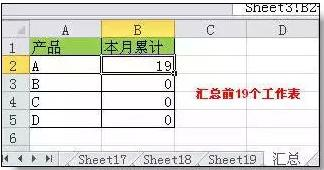6. 按日期和产品求和

(七)查找与引用公式

1. 单条件查找公式

公式1： C11=VLOOKUP(B11,B3:F7,4,FALSE)

说明：查找是VLOOKUP最擅长的，基本用法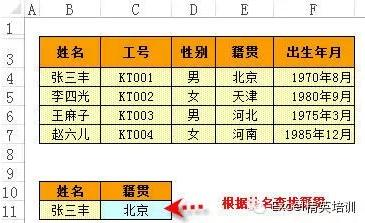2. 双向查找公式

公式=INDEX(C3:H7,MATCH(B10,B3:B7,0),MATCH(C10,C2:H2,0))

说明：利用MATCH函数查找位置，用INDEX函数取值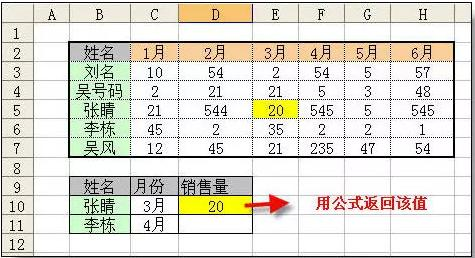3. 查找最后一条符合条件的记录。

公式：详见下图

说明：0/(条件)可以把不符合条件的变成错误值，而lookup可以忽略错误值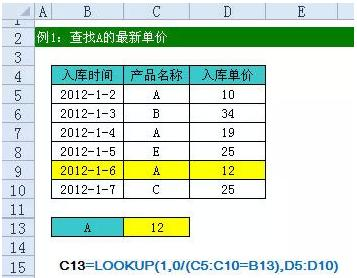4. 多条件查找

公式：详见下图

说明：公式原理同上一个公式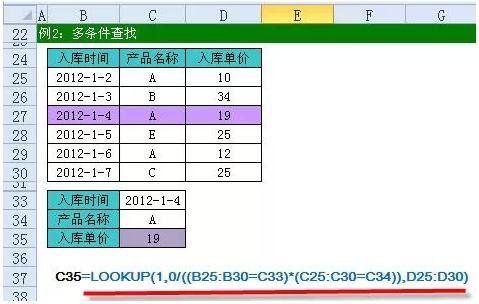5. 指定区域最后一个非空值查找

6. 按数字区域间取对应的值

展开全文•excel 函数
• 包含500多种函数常用公式汇总教学，需要多种函数支持，适合办公人士和财务制表常用公式，商务会谈，数据报表制表。
• 从英文名称的中文含义出发，循序渐进、由浅入深，带领大家轻松了解和掌握工作中最为常用的33个函数。适合：迫切想提高工作效率的职场人、EXCEL小白、数据或文字工作者等。Office/WPS
• 对于想学习数据分析的同学来说还是不错的，主要讲解了一些关于Excel常用函数
• Excel函数公式大全（史上最全最新2022年版，482个函数）,适合excel新手学习使用，同时也适合高手查阅函数excel新增函数标注了适用版本。
• EXCEL常用函数公式与举例.pdfEXCEL常用函数公式与举例.pdfEXCEL常用函数公式与举例.pdfEXCEL常用函数公式与举例.pdfEXCEL常用函数公式与举例.pdf
• ## excel常用函数大全

千次阅读 2021-06-28 13:43:15
excel是一款非常好用的表格数据处理软件，在这款软件中有着大量的函数功能来帮我们进行数据处理，可以让我们能够简单的进行大批量的数据计算，那么excel常用函数有哪些呢？excel常用函数大全1、SUM函数：SUM函数的...

excel是一款非常好用的表格数据处理软件，在这款软件中有着大量的函数功能来帮我们进行数据处理，可以让我们能够简单的进行大批量的数据计算，那么excel常用函数有哪些呢？

excel常用函数大全

1、SUM函数：SUM函数的作用是求和。函数公式为=sum()

例如：统计一个单元格区域：=sum(A1:A10) 统计多个单元格区域：=sum(A1:A10,C1:C10)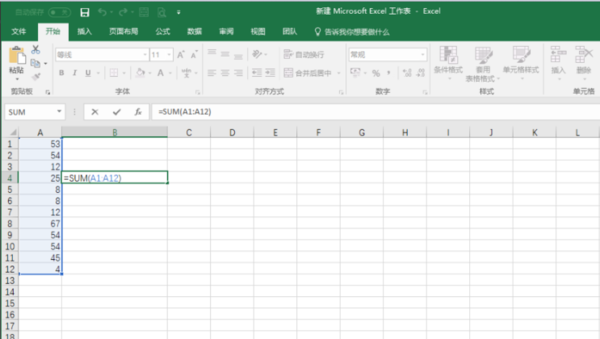2、AVERAGE函数:Average 的作用是计算平均数。函数公式为=AVERAGE( )

例如：计算多个单元格的平均值=AVERAGE(A1:A12)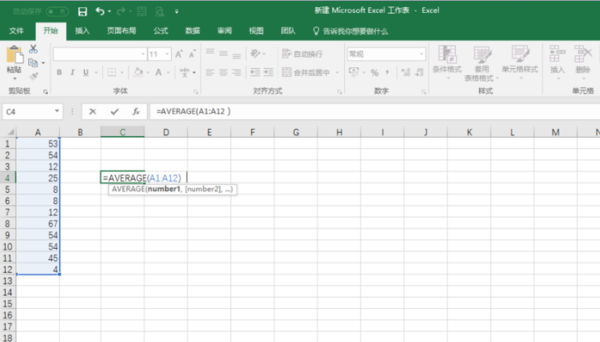3、count函数：作用是计算单元格个数 。函数公式为=COUNT( )

例如=COUNT(A1:A12)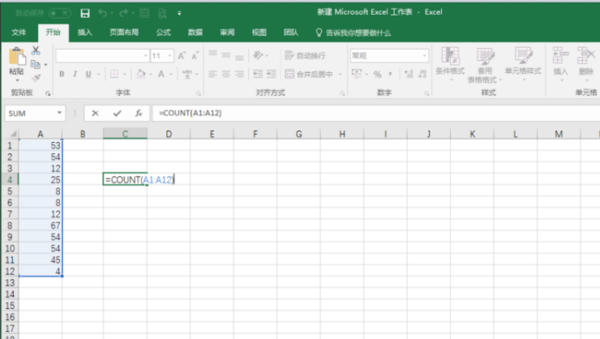4、IF函数：IF函数的作用是判断一个条件，然后根据判断的结果返回指定值。

IF函数公式为：=IF(逻辑判断,为TRUE时的结果,为FALSE时的结果)

例如：给出的条件是A1>A5，如果比较结果是TRUE，那么IF函数就返回第二个参数的值;如果是FALSE，则返回第三个参数的值。=IF(A1>A5,1,2)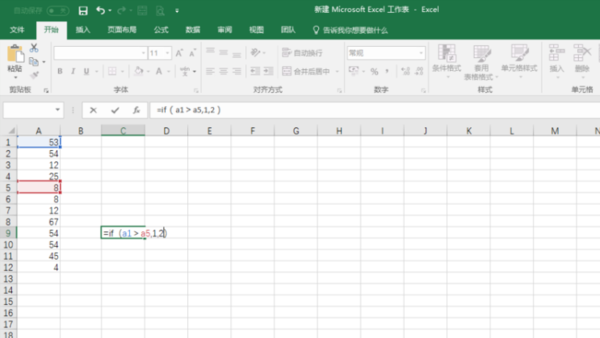5、NOW函数和TODAY函数：NOW函数返回日期和时间。TODAY函数则只返回日期。

NOW函数和TODAY函数都没有参数，只用一对括号即可：=NOW()=TODAY()

假如说，要计算某项目到今天总共进行多少天了?=TODAY()-开始日期，得出的数字就是项目进行的天数。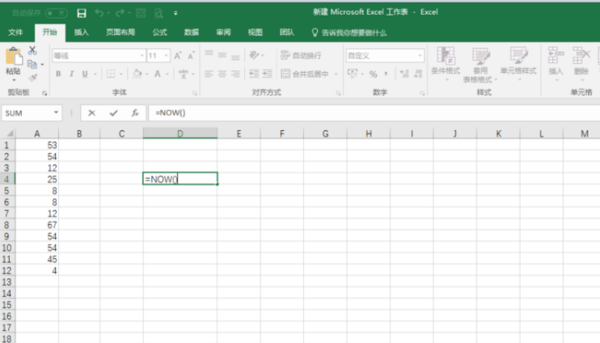6、VLOOKUP函数：VLOOKUP函数用来在表格中查找数据。

函数的语法公式是：=VLOOKUP(查找值,区域,要返回第几列的内容,1近似匹配 0精确匹配)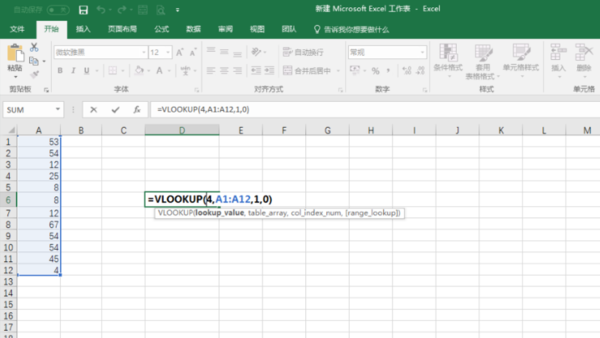以上就是excel常用函数大全。

展开全文•Excel函数
• 在学习python的时候写的用python来处理excel表格的20个函数（包括批量提取表格数据，批量处理表格数据等），内含详细注释和说明，可用于python自动化办公入门学习。
• 使用工具：Microsoftoffice excel 2016 一、Vlookup函数。 功能：查找引用。 语法：=Vlookup(查找值，查找范围，返回查找范围中的第几列，匹配模式)。 方法：在目标单元格中输入公式：=VLOOKUP(H3,B3:D9,3,0)。 ...

## 使用工具：Microsoft office excel 2016

目录

一、Vlookup函数。

二、Sumif函数。

三、Countif函数。

四、If函数。

五、Sumifs函数。

六、Countifs函数。

七、Round和Int函数。

八、Left、Mid、Right函数。

九、Datedif函数。

十、Iferror函数。

十一、Average和Averageif函数。

十二、Year、Month、Day函数。

十三、Date函数。

十四、Index函数。

十五、Len、Lenb函数。

十六、Match函数。

十七、Mod函数。

十八、Today和Now函数。

十九、Rank函数。

二十、Text函数。

### 一、Vlookup函数。

功能：查找引用。

语法：=Vlookup(查找值，查找范围，返回查找范围中的第几列，匹配模式)。

方法：在目标单元格中输入公式：=VLOOKUP(H3,B3:D9,3,0)。

解读：

① 返回B3：D9范围中和H3相匹配的第3列的值。

② 匹配模式有2种，1为模糊匹配，0位精准匹配。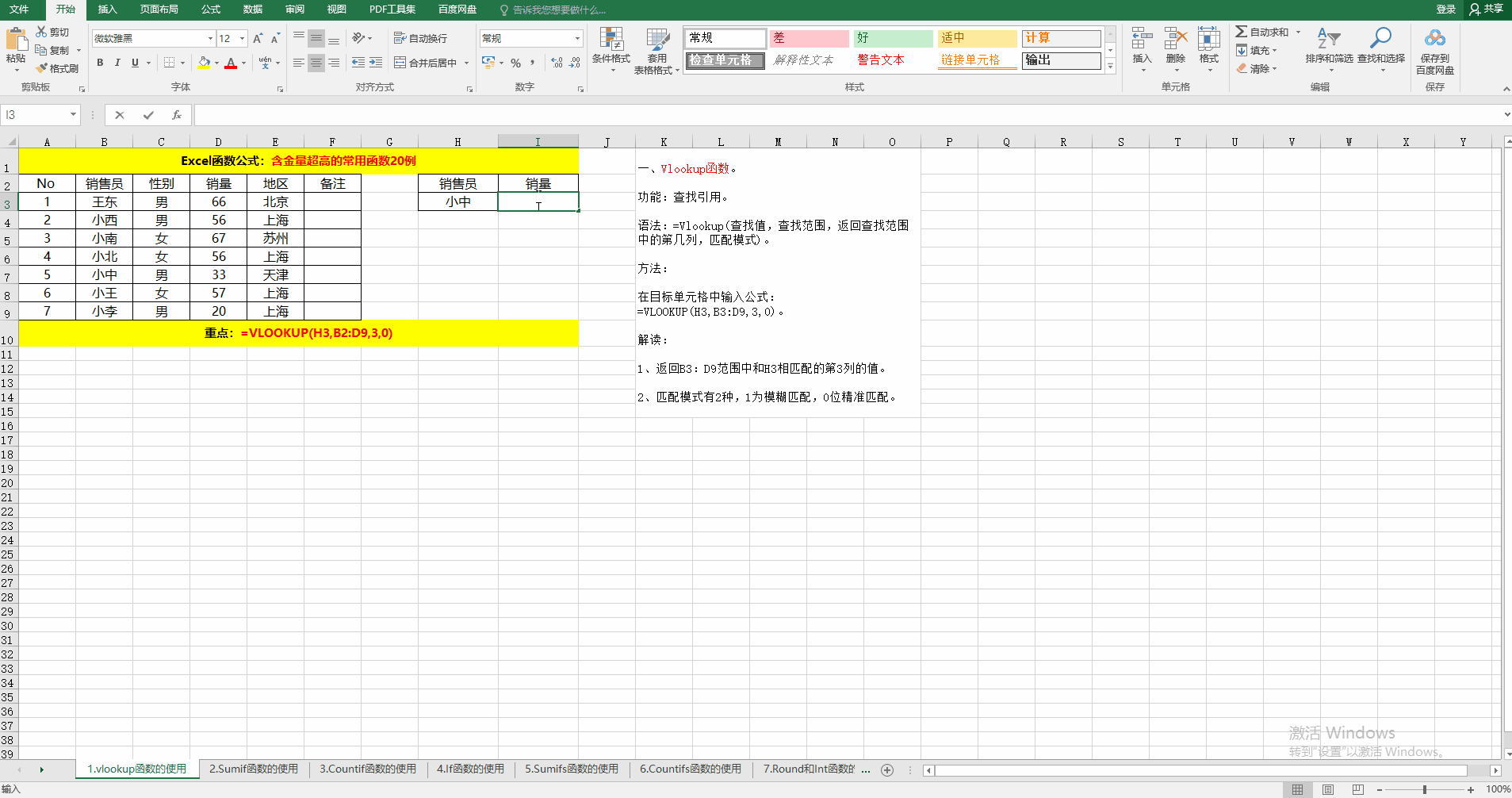### 二、Sumif函数。

功能：单条件求和。

语法：=Sumif（条件范围，条件，求和范围）。

方法：在目标单元格中输入公式：=SUMIF(C3:C9,H3,D3:D9)。

解读：求和的目的在于：统计男or女销售员的销量和。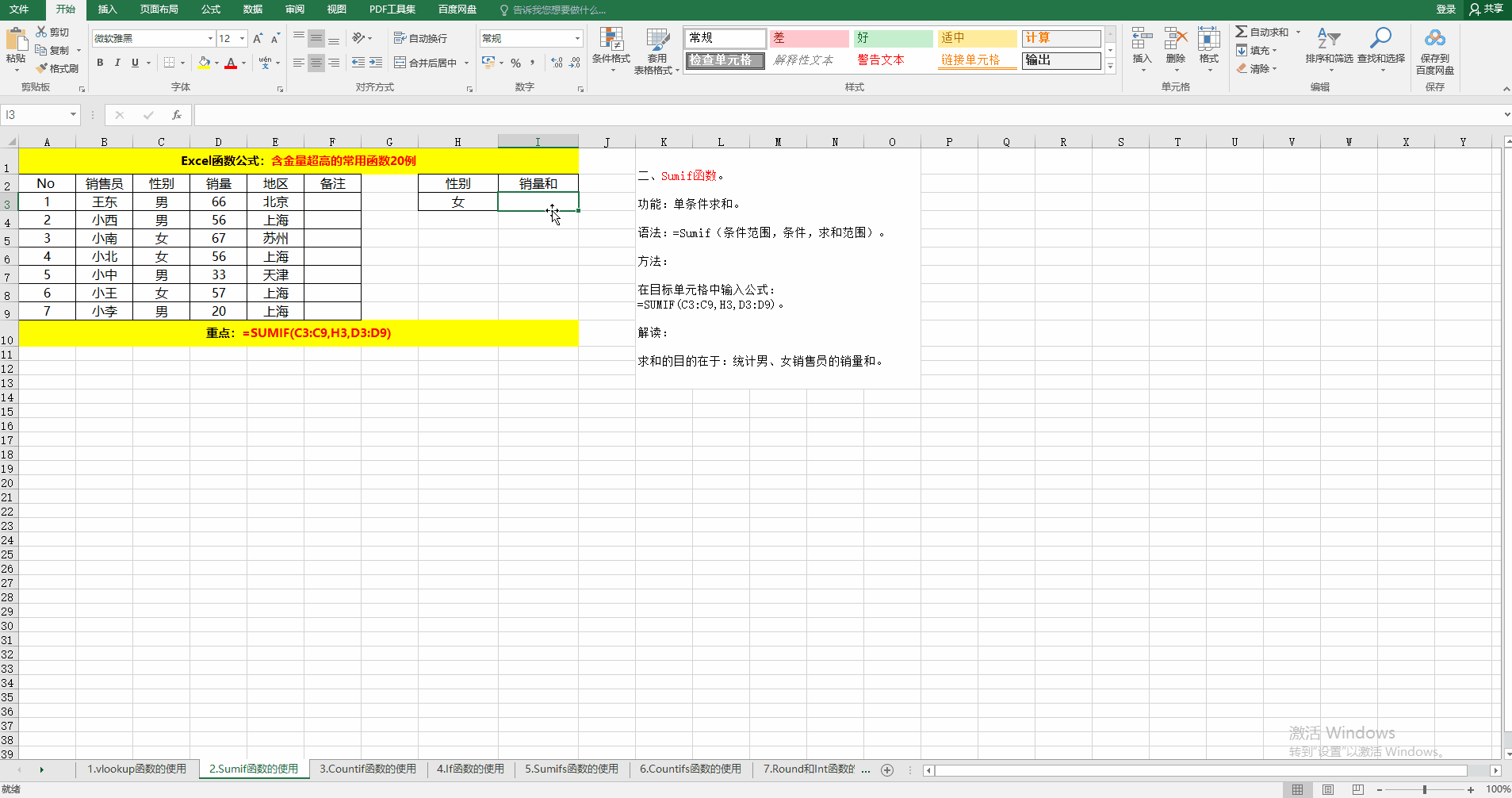### 三、Countif函数。

功能：条件计数。

语法：=Countif(条件范围，条件)。

方法：在目标单元格中输入公式：=COUNTIF(C3:C9,H3)。

解读：示例的目的在于统计男or女销售员的人数。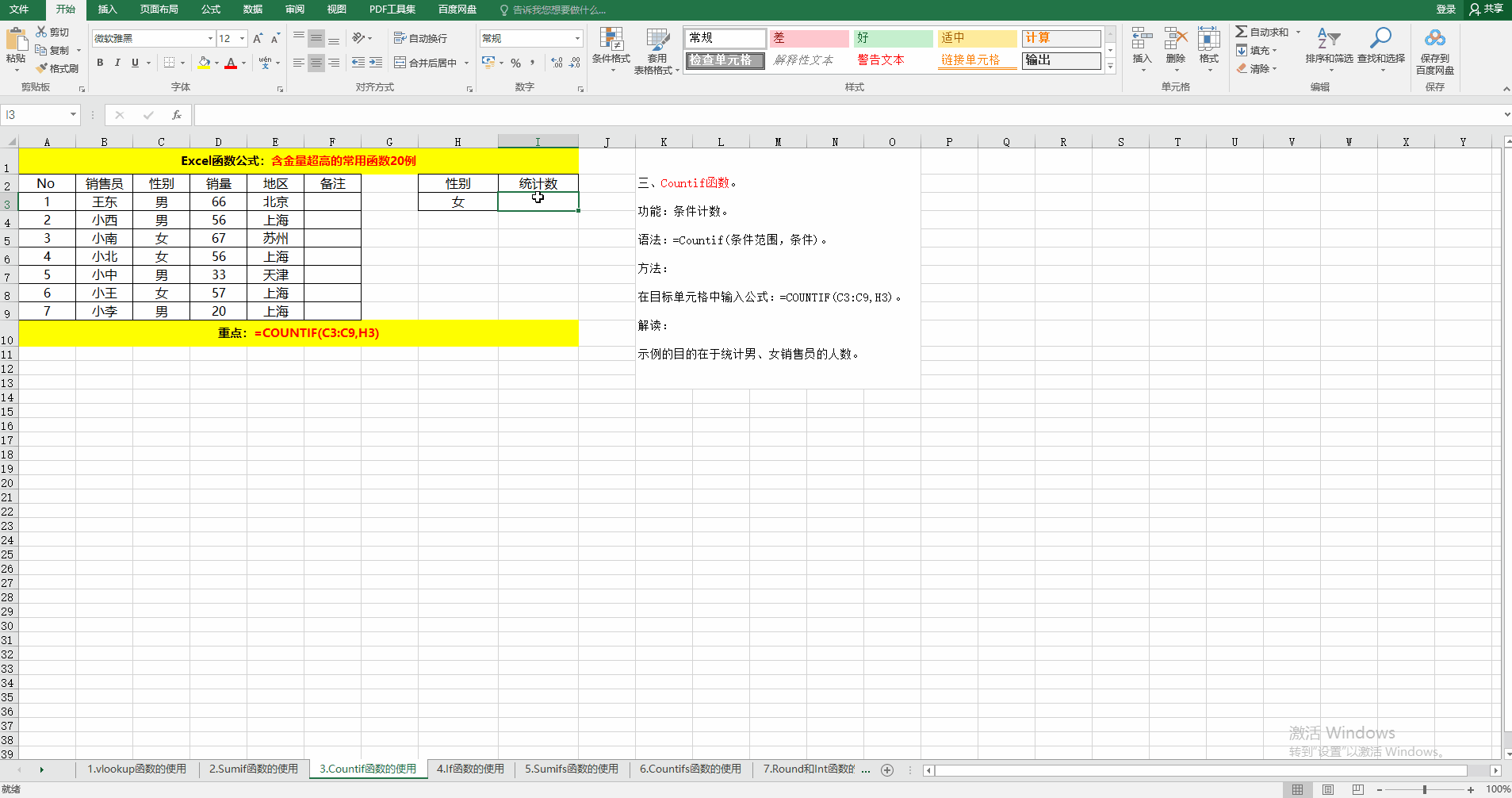### 四、If函数。

功能：根据条件判断结果返回相应的值或公式的计算结果。

语法：=If(判断条件，条件为真时返回的值，条件为假时返回的值）。

方法：在目标单元格中输入公式：=IF(D3>=60,"是","否")。

解读：如果销量大于等于60，则返回"是"，否则返回"否"。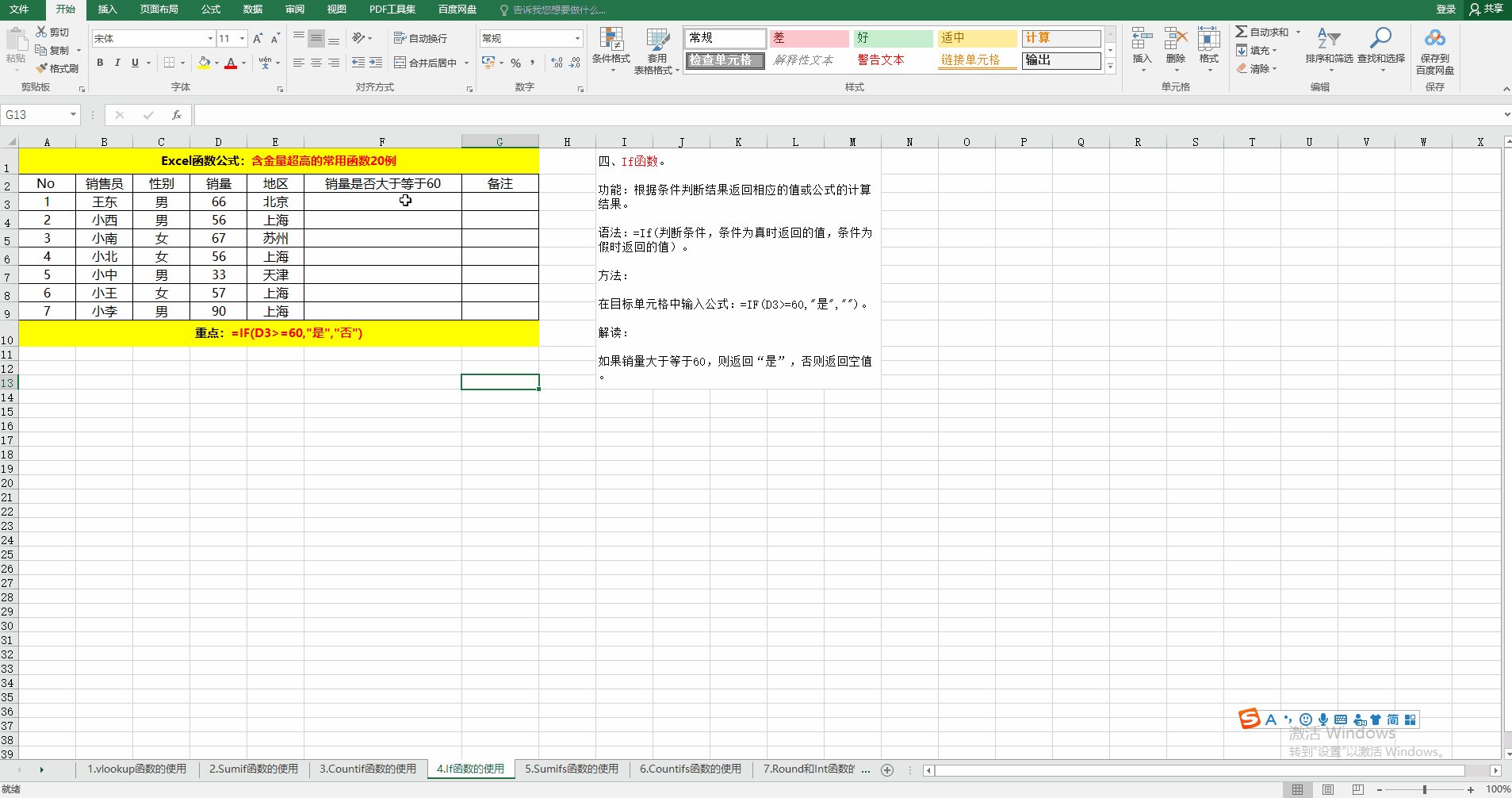### 五、Sumifs函数。

功能：多条件求和。

语法：=Sumifs(求和范围，条件1范围，条件1,……条件N范围，条件N)。

方法：在目标单元格中输入公式：=SUMIFS(D$3:D$9,C$3:C$9,H3,D$3:D$9,I3)。

解读：求男、女销售员销量大于等于60的销量和。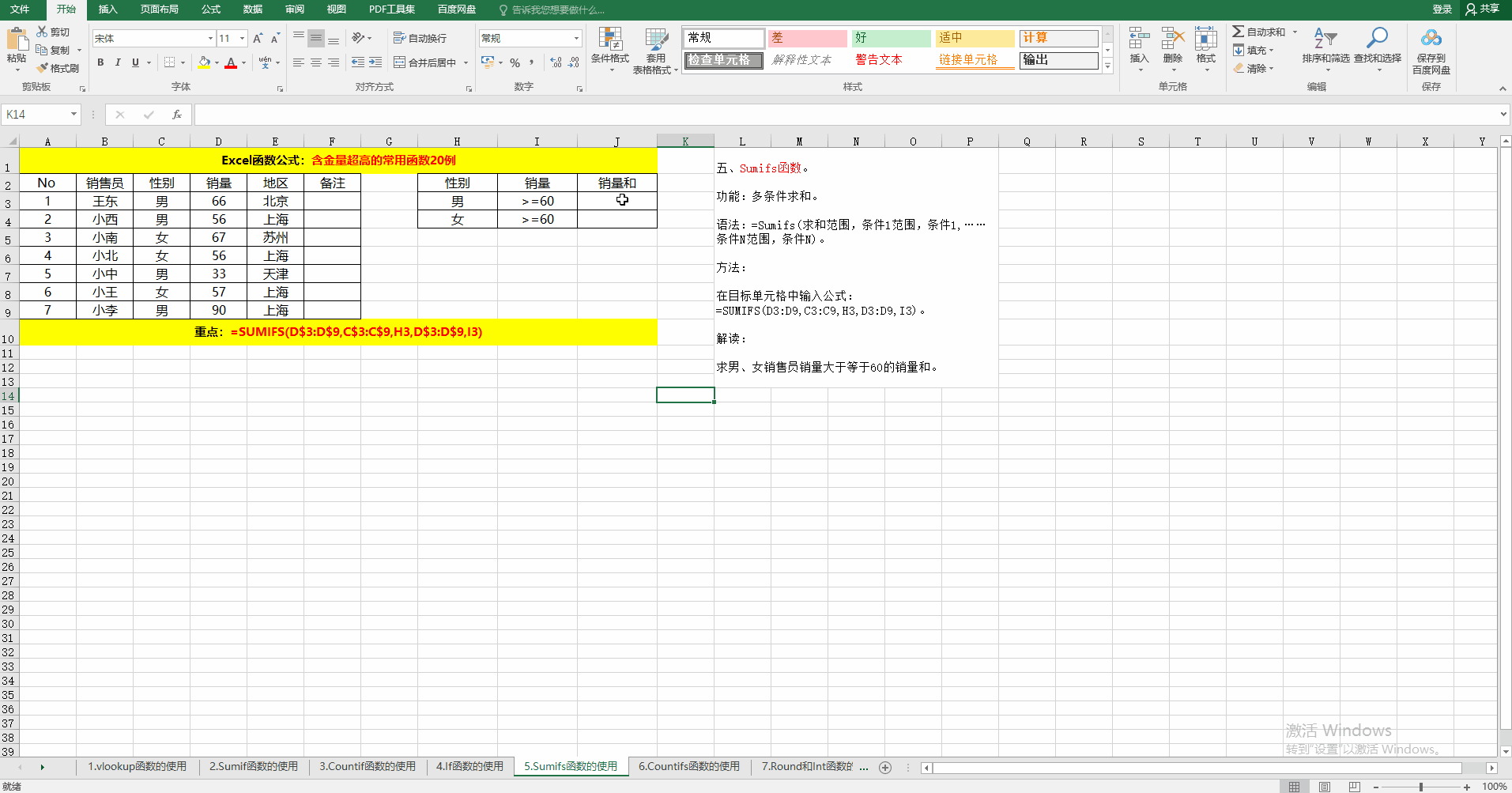### 六、Countifs函数。

功能：多条件计数。

语法：=Countifs(条件1范围，条件1……条件N范围，条件N）。

方法：在目标单元格中输入公式：=COUNTIFS(C$3:C$9,H3,D$3:D$9,I3)。

解读：求男、女销售员销量大于等于60的人数。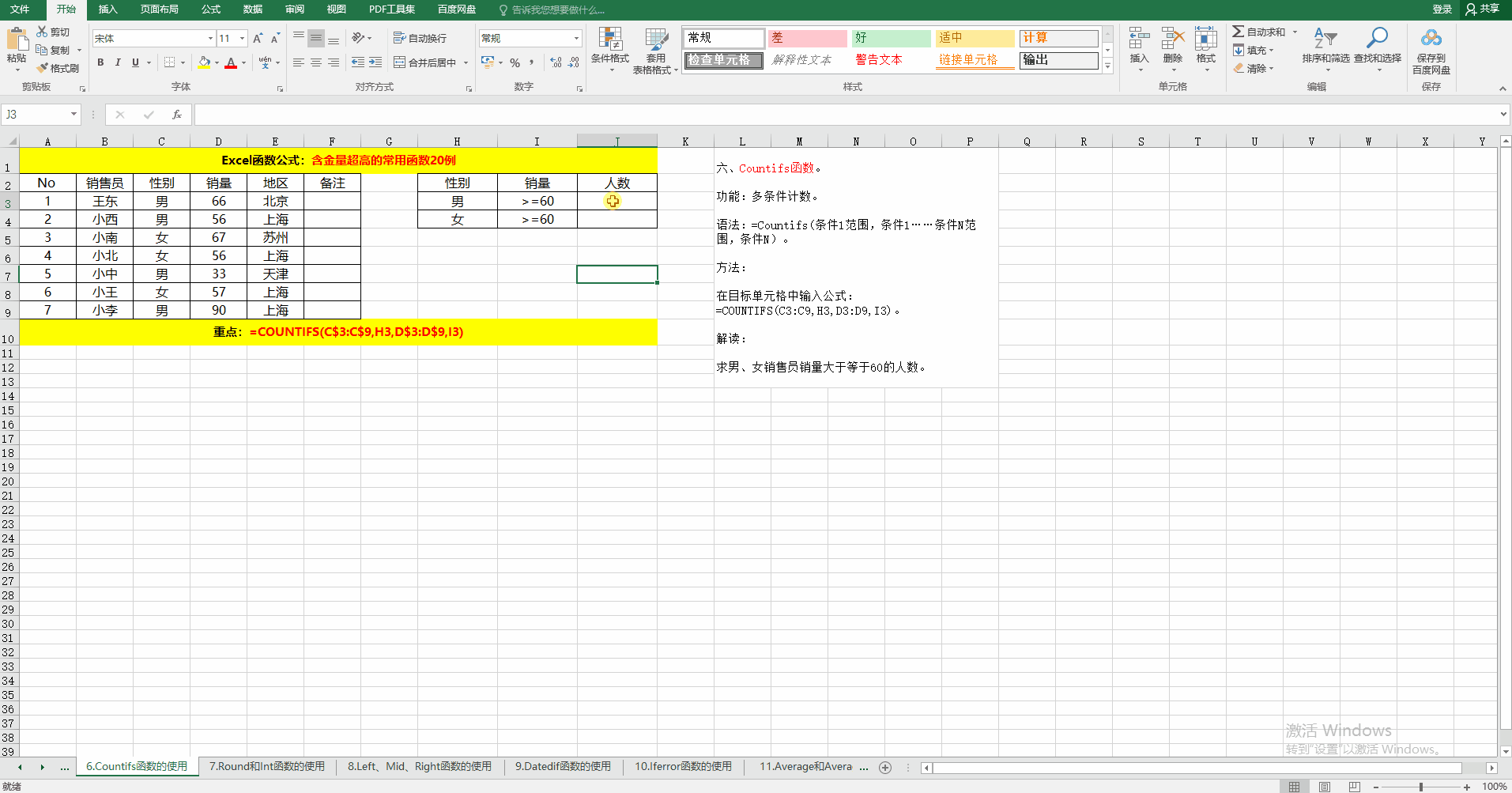### 七、Round和Int函数。

功能：四舍五入、取整。

语法：=Round（值，保留位数）；=Int（值）。

方法：在目标单元格中输入公式：=ROUND(I3,1)、=INT(I3)。

解读：Round函数根据保留位数四舍五入。而Int函数直接取整数位，不进行四舍五入。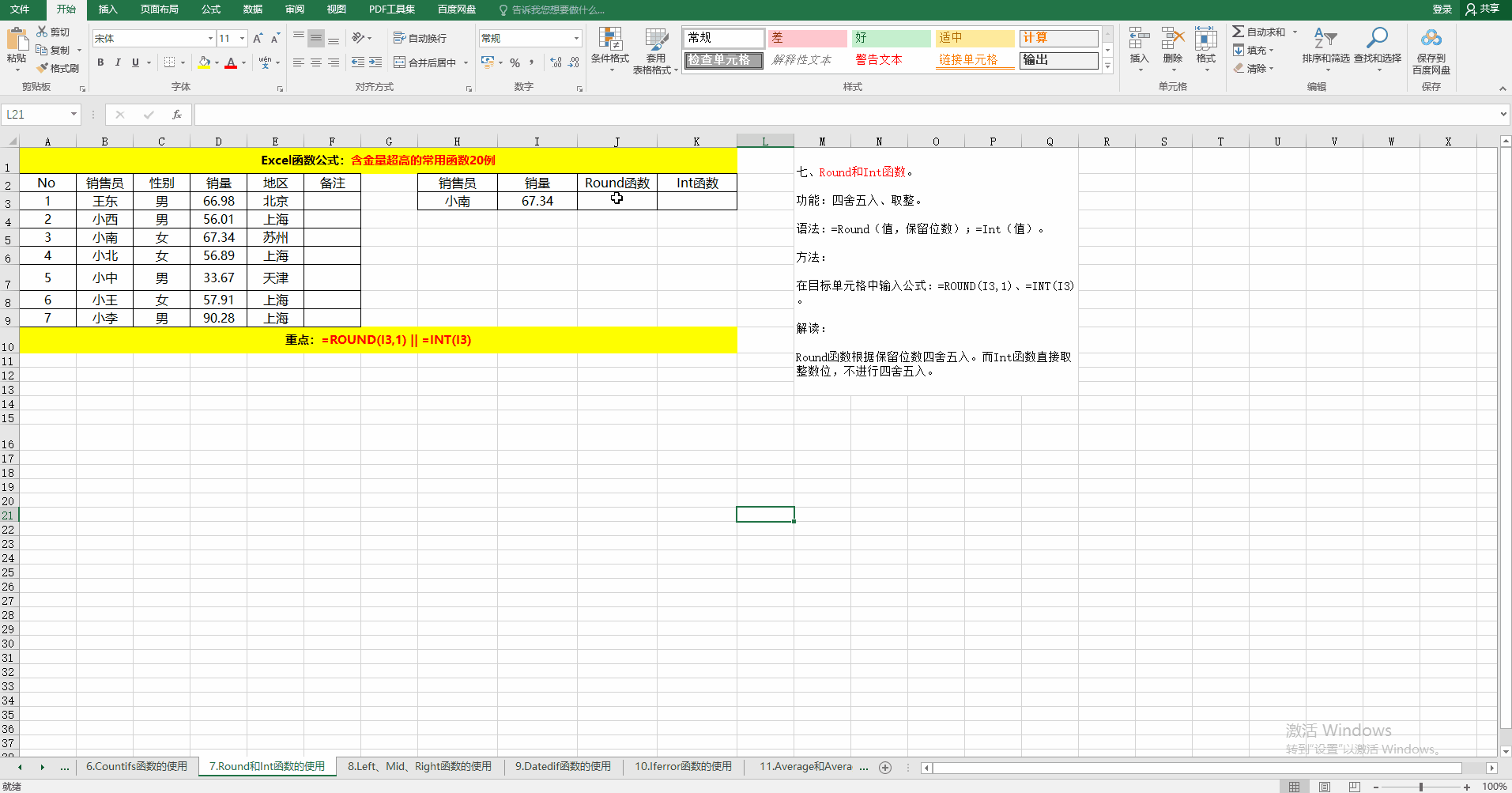### 八、Left、Mid、Right函数。

功能：提取指定的字符串。

语法：

① =Left(字符串，从左边开始提取的字符串位数)。

② =Mid(字符串，开始字符串的位置，需要截取的长度)。

③ =Right(字符串，从右边开始提取的字符串位数)。

方法：在目标单元格中输入公式：=LEFT(F3,4)、=MID(F3,5,5)、=RIGHT(F3,4)。

解读：从指定的字符串中提取指定的字符串。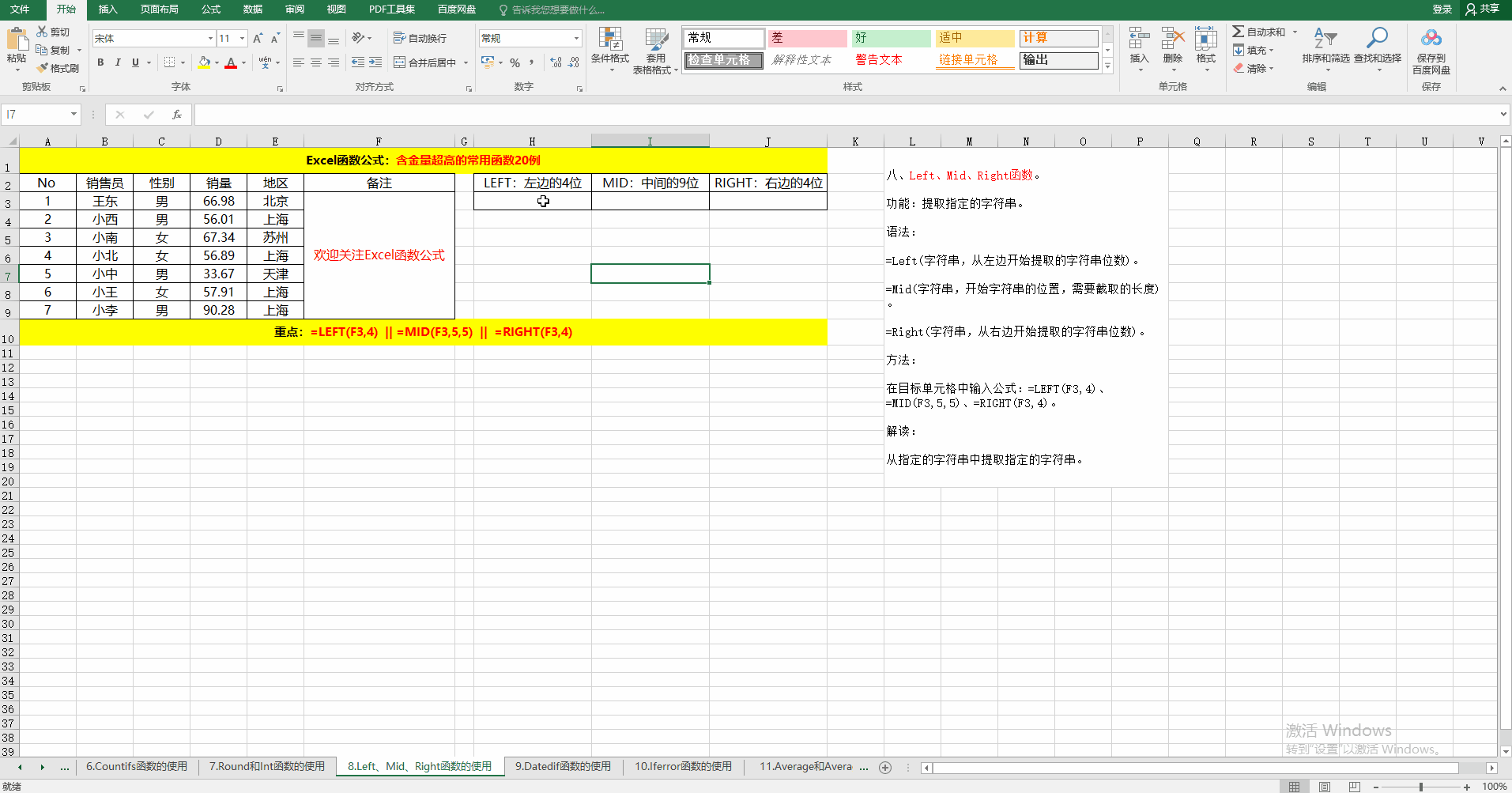### 九、Datedif函数。

功能：按指定的统计方式统计时间差。

语法：=Datedif(开始时间，结束时间，统计方式)。

方法：在对应的目标单元格中输入公式：=DATEDIF(C3,D3,"y")、=DATEDIF(C3,D3,"m")、=DATEDIF(C3,D3,"d")。

解读：Datedif函数为系统隐藏函数，其统计方式有：Y（年）、M（月）、D（日）、MD（忽略年和日的天数）、YM（忽略年和日的月数）、YD（忽略年的日的天数）。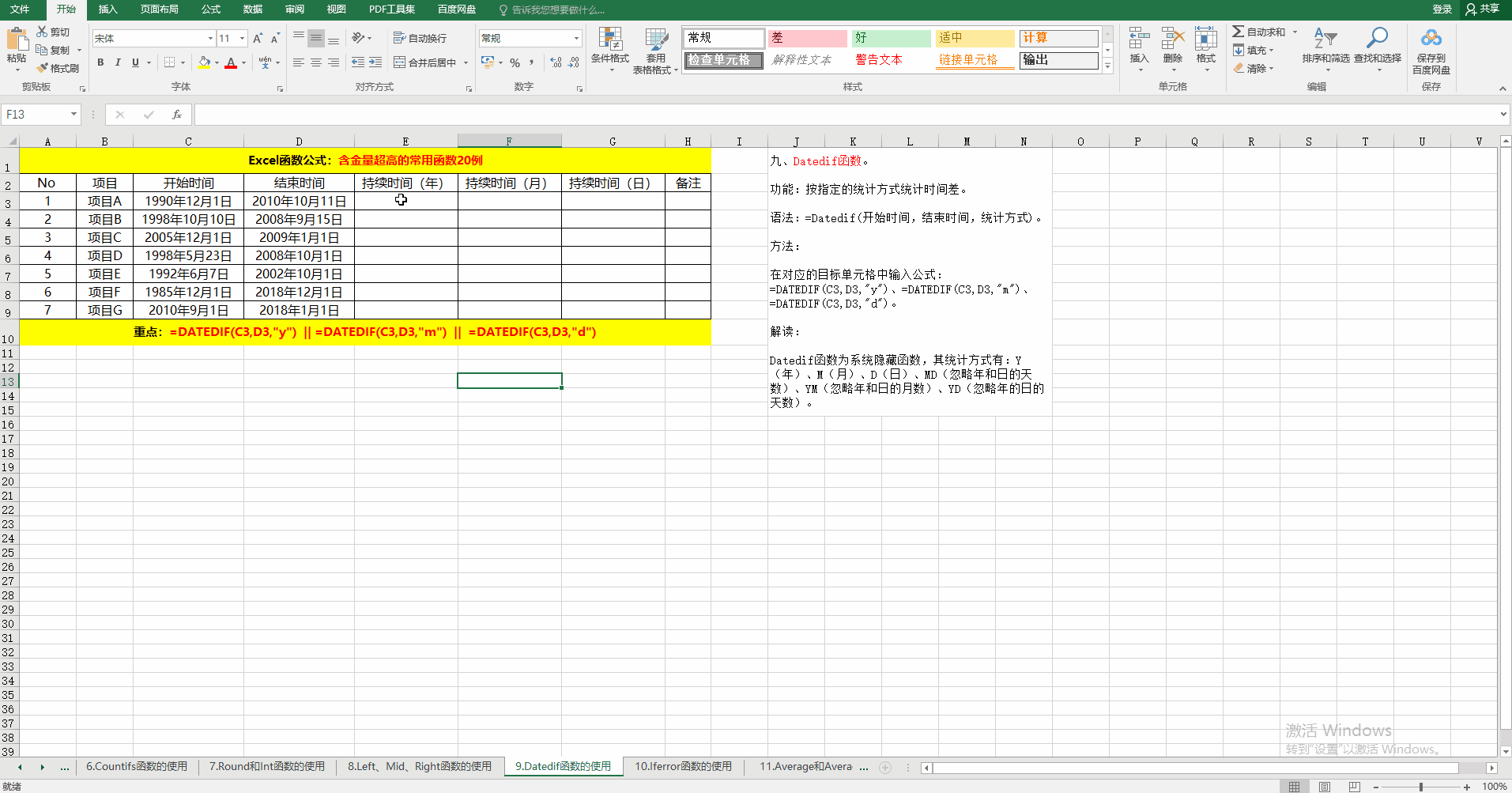### 十、Iferror函数。

功能：判断表达式或公式是否有错误，如果有错误，则返回指定的值，否则返回表达式或公式本身的值。

语法：=Iferror(表达式或公式，公式错误时返回的值)。

解读：除数不能为0，当除数为0时返回错误。利用Iferror返回空值。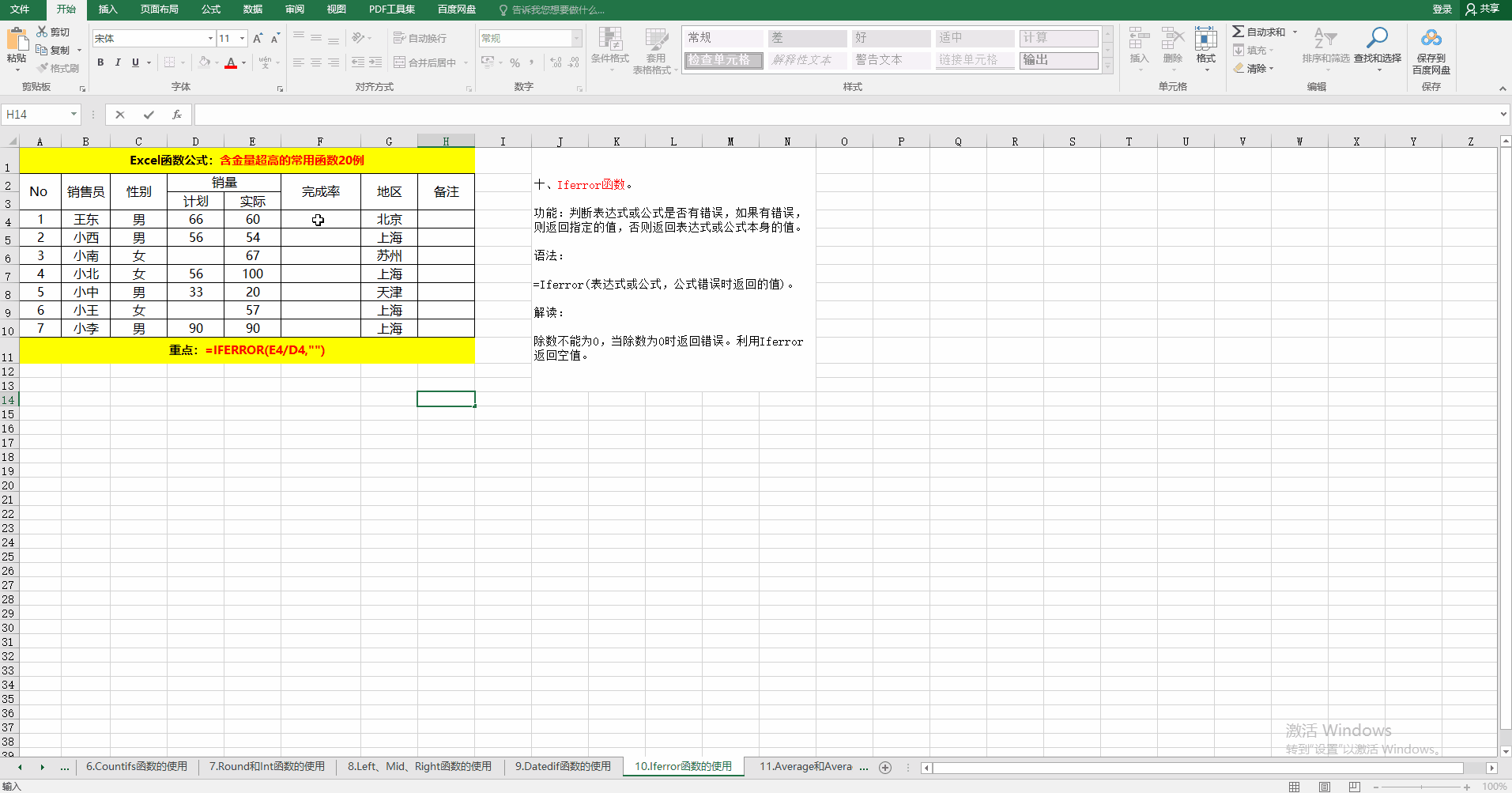### 十一、Average和Averageif函数。

功能：计算平均值或根据指定的条件计算平均值。

语法：

① =Average(值范围)。

② =Averageif(条件范围，条件，值范围）。当条件范围和值范围相同时，值范围可以省略哦！

方法：在目标单元格中输入公式：=AVERAGE(D3:D9)、=AVERAGEIF(C3:C9,H4,D3:D9)。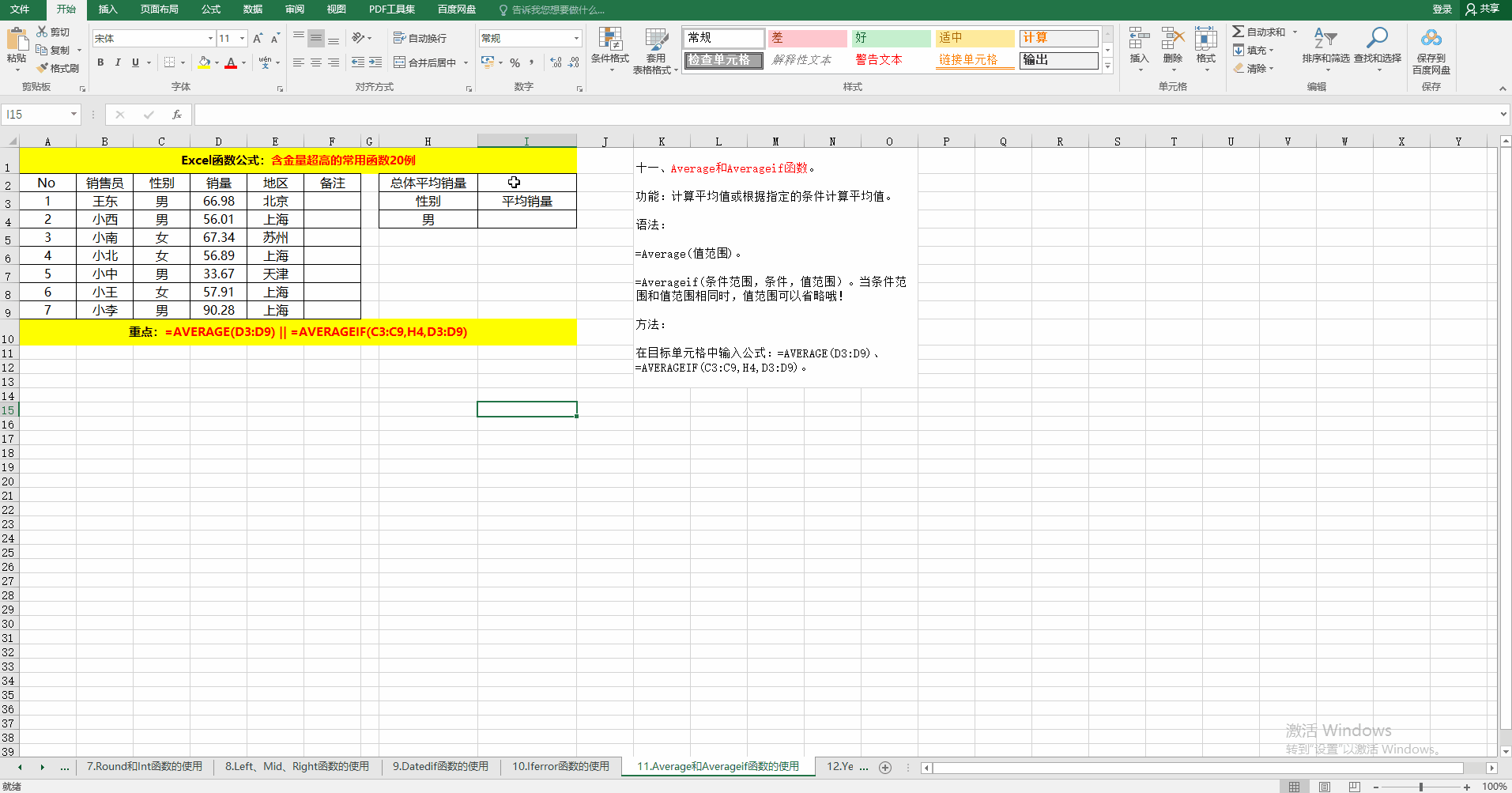### 十二、Year、Month、Day函数。

作用：提取日期中的年（Year）、月（Month）、日（Day）。

语法：=Year（日期）；=Month（日期）；=Day（日期）。

方法：在目标单元格中输入公式：=YEAR(C3)、=MONTH(C3)、=DAY(C3)。

解读：利用函数Year、Month、Day可以提取日期中对应的值，也可以利用Date将数值组成日期。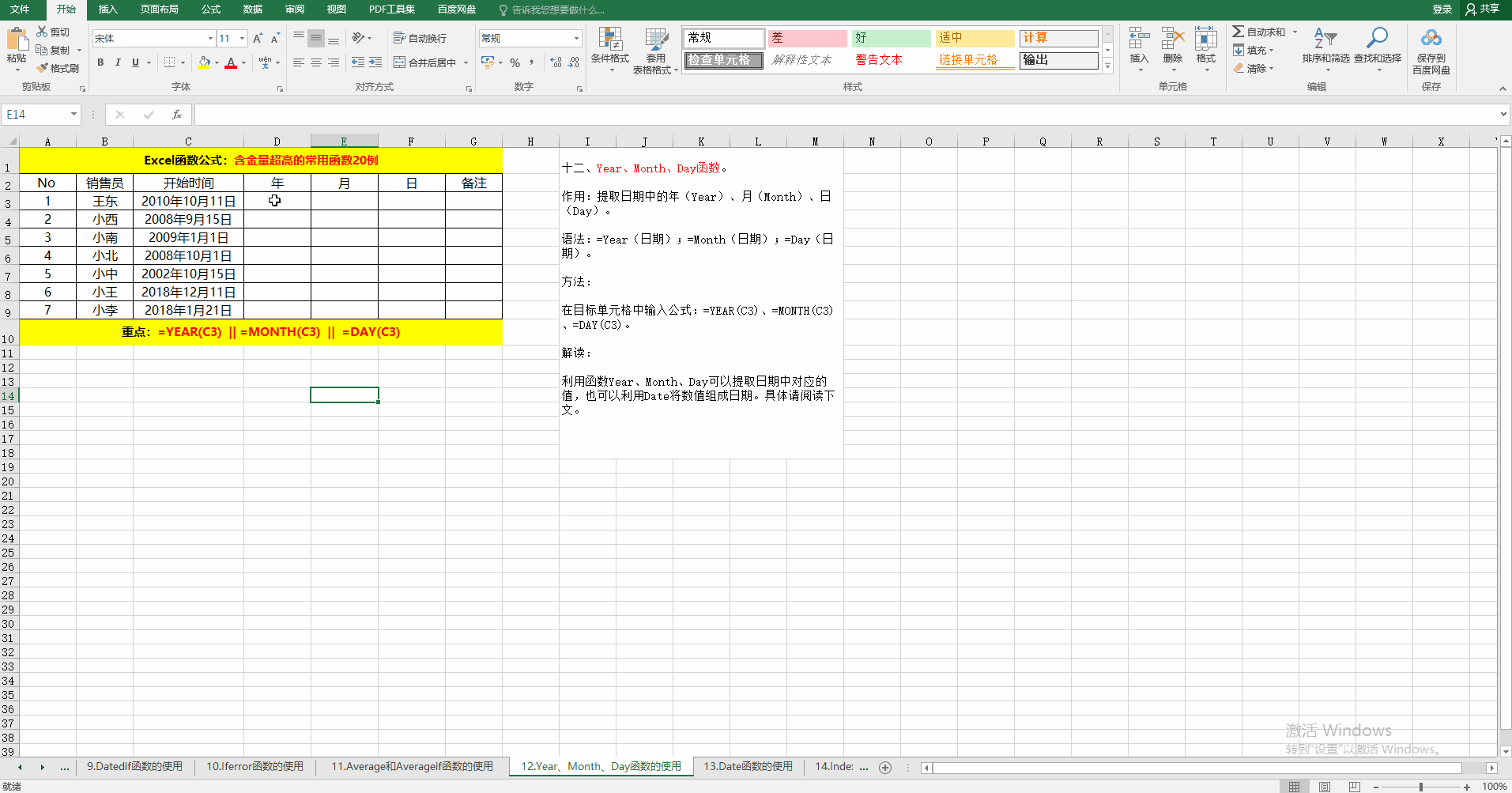### 十三、Date函数。

作用：将指定的值转换为日期。

语法：=Date（表示年的值，表示月的值，表示日的值）。

方法：在目标单元格中输入公式：=DATE(C3,D3,E3)。

解读：

①未转换之前单元格的格式为“常规”。

②转换后单元格的格式变为“日期”，自动变化哦！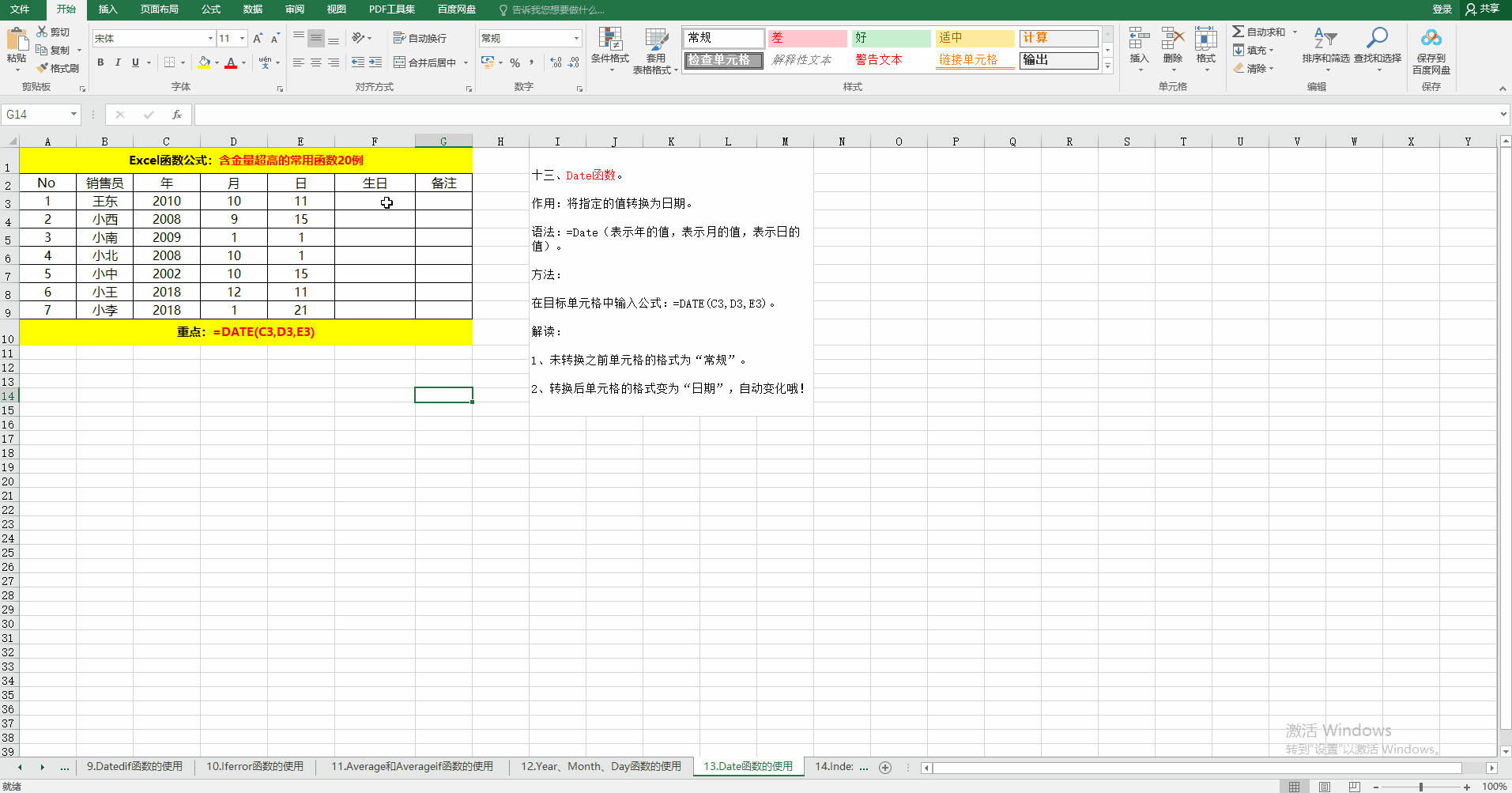### 十四、Index函数。

作用：返回列表或数组中指定位置的值。

语法：=Index(数据区域，行，列)。

方法：在目标单元格中输入公式：=INDEX(B3:E9,3,4)。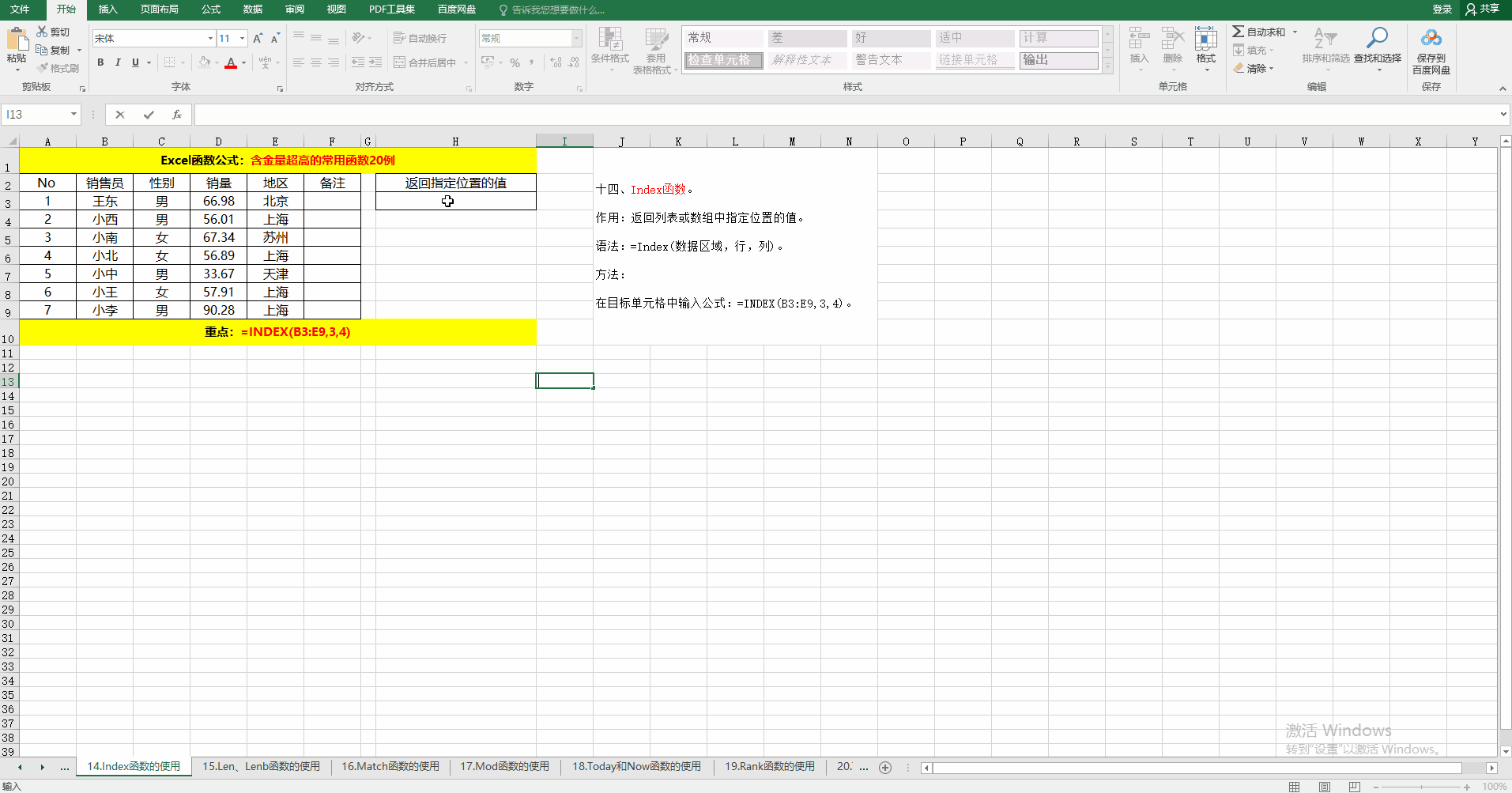### 十五、Len、Lenb函数。

作用：返回字符串中的字符串和字节数。

语法：=Len(字符串)、=Lenb(字符串)。

方法：在目标个输入公式：=LEN(A1)、=LENB(A1)。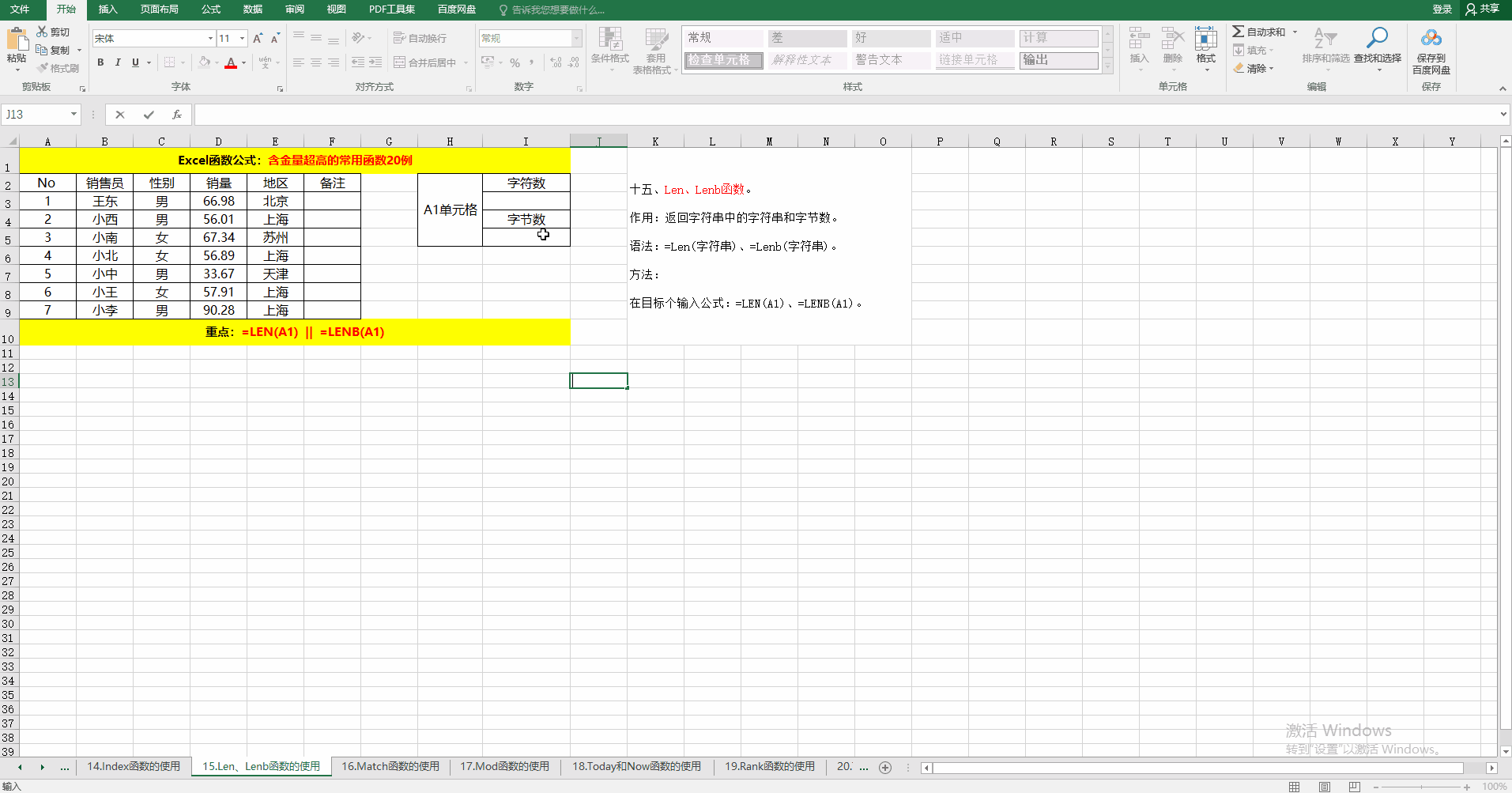### 十六、Match函数。

作用：返回指定方式下与指定值匹配的数组元素中元素的位置。

语法：

=Match(查找值，查找值所在的返回，匹配方式)。

其中匹配方式为：-1,0,1。其所表示的意义分别为：

-1：查找大于或等于查找值的最小值，查找范围必须按降序排列。

0：精准查找，查找范围可以按任何顺序排列，如果省略第三个参数，默认为0.

1：查找小于或等于查找值的最大值，查找范围必须按升序排列。

方法：在目标单元格中输入公式：=MATCH(H3,B3:B9,0)。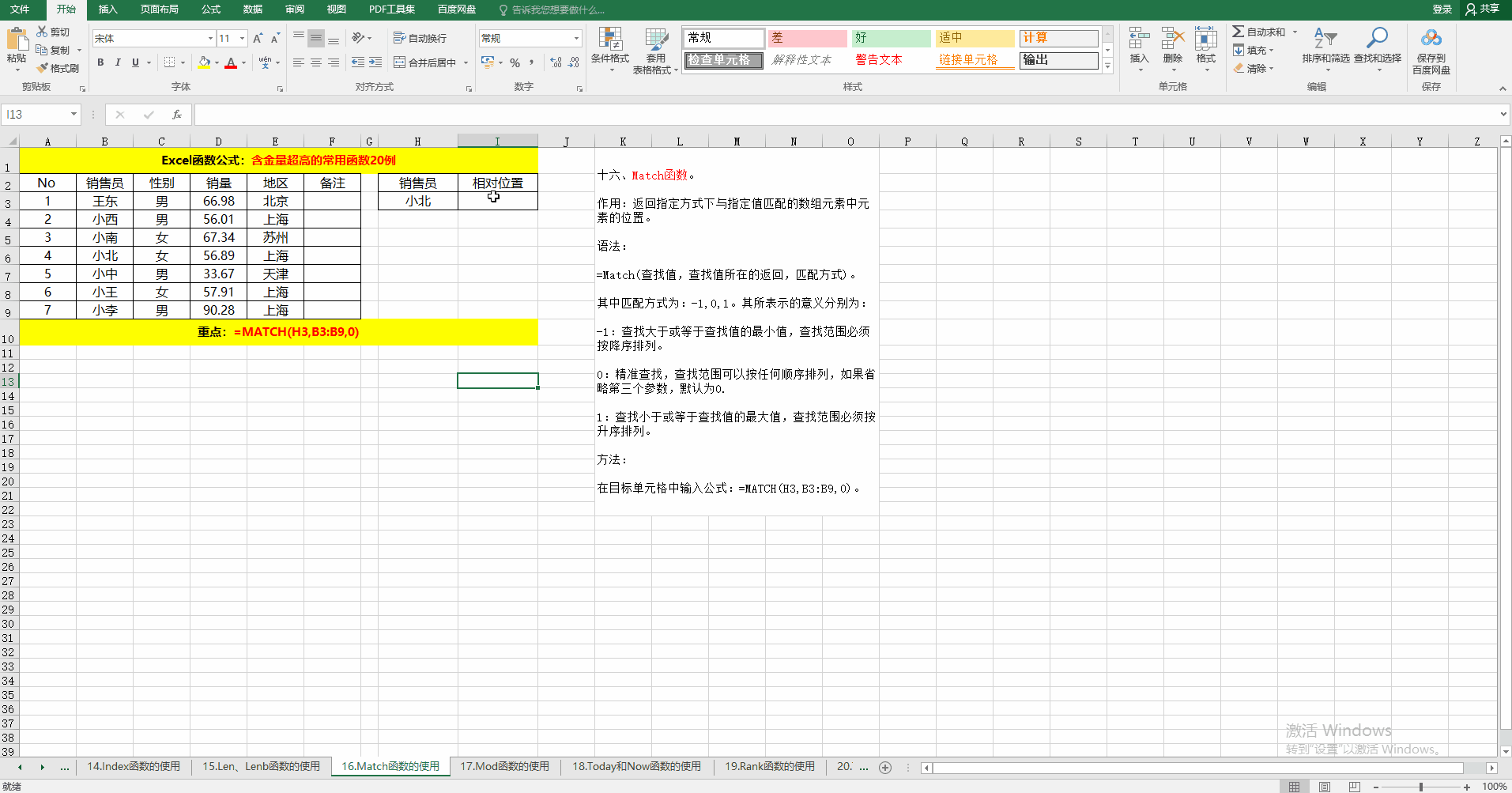### 十七、Mod函数。

作用：计算两个数相除之后的余数。

语法：=Mod(被除数，除数)。

方法：在目标单元格中输入公式：=MOD(B3,C3)。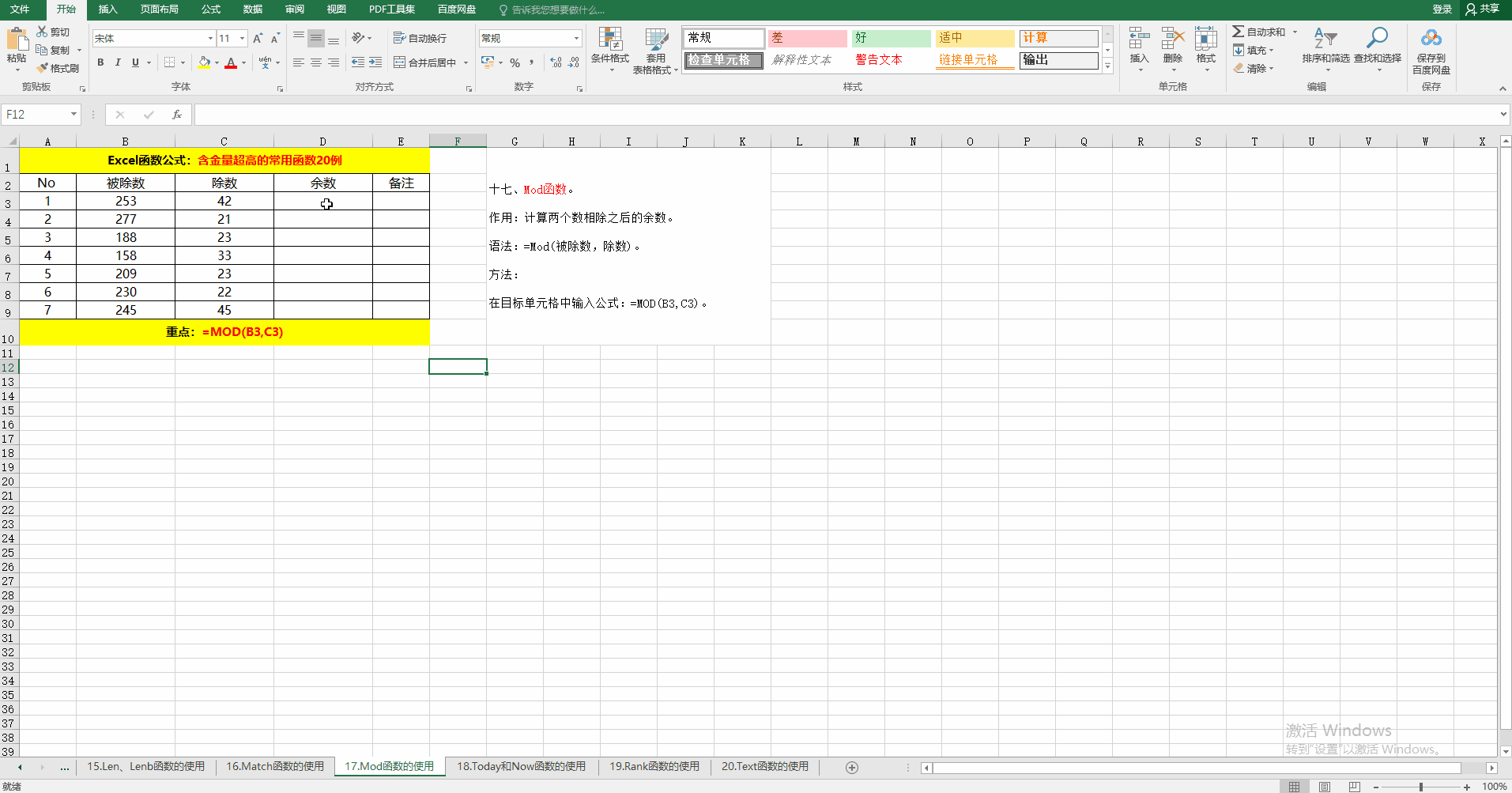### 十八、Today和Now函数。

作用：获取系统当前日期和时间。

语法：=Today() 、=Now()。

方法：在目标单元格中输入公式或按相应的快捷键。

解读：获取系统日期和时间除了用函数之外，还可以实用快捷键，分别为：Ctrl + ; 和 Ctrl+Shift + ; 。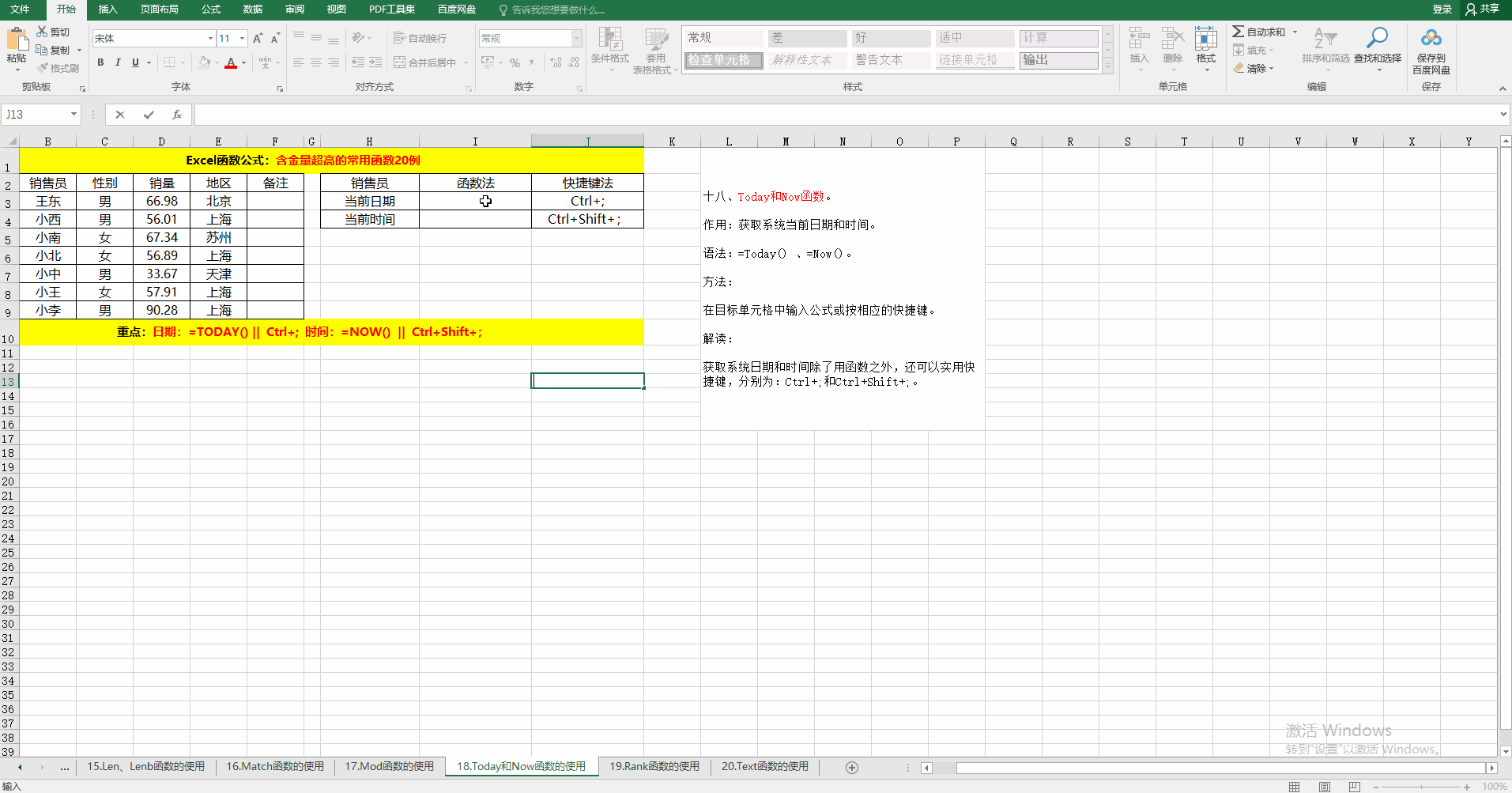### 十九、Rank函数。

作用：对给定的值按照指定的顺序排序。

语法：=Rank(排序的值，排序范围，排序方式)。

排序方式有1和0两种，1为升序，0位默认值，降序。

方法：

在目标单元格中输入公式：

① 升序=RANK(D3,D$3:D$9,1)

② 降序=RANK(D3,D$3:D$9,0)或=RANK(D3,D$3:D$9)。

解读：当省略第三个参数时，默认为降序排序，和第三个参数为0的效果相同哦！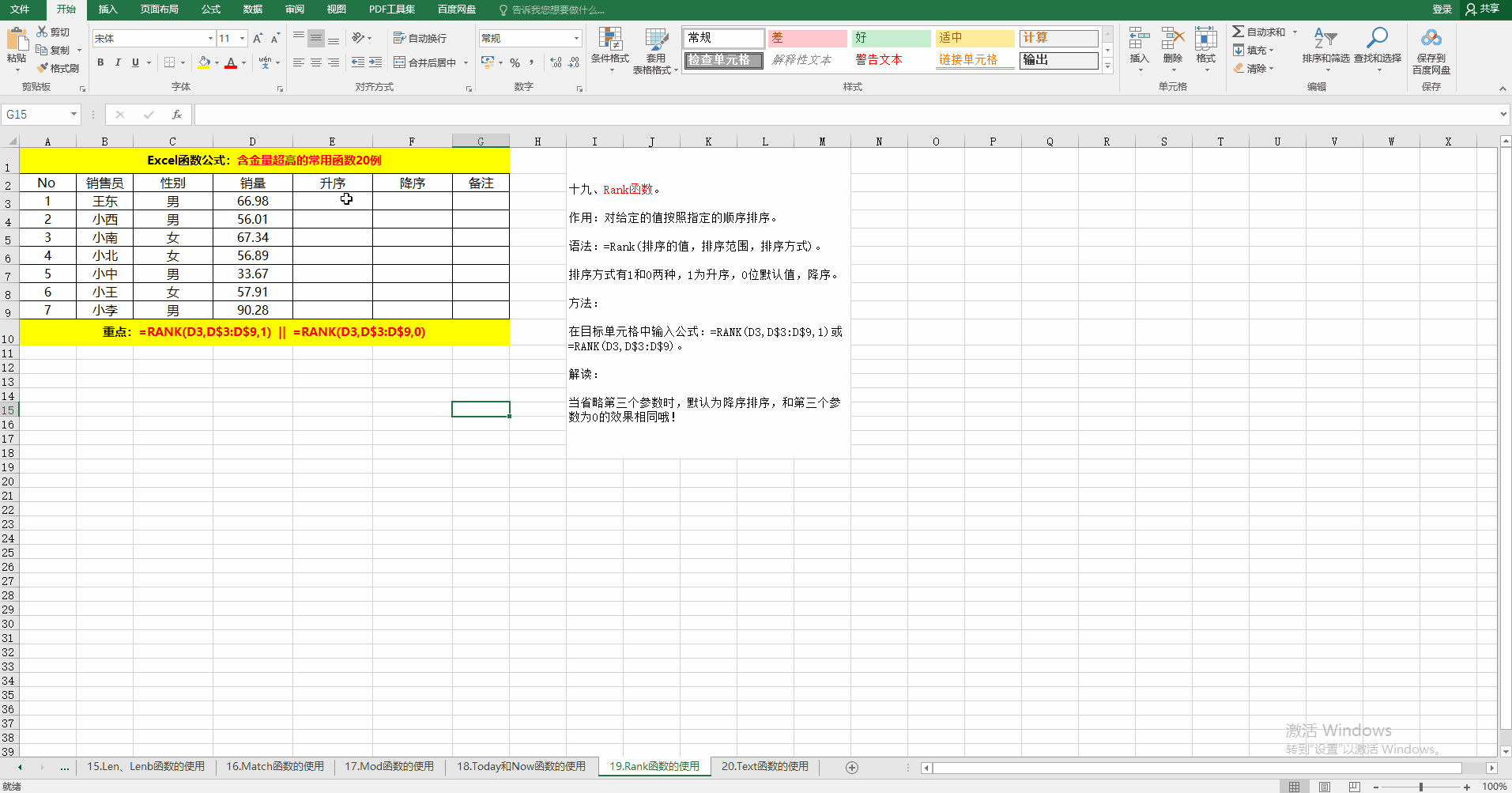### 二十、Text函数。

作用：根据指定的代码将相应的值设置为相应的格式。

语法：=Text(值，格式)。

方法：在目标单元格中输入公式：=TEXT(D3,"\$0.00")。

解读：示例中将数字转换为货币形式，当然还可以转换为其它形式哦。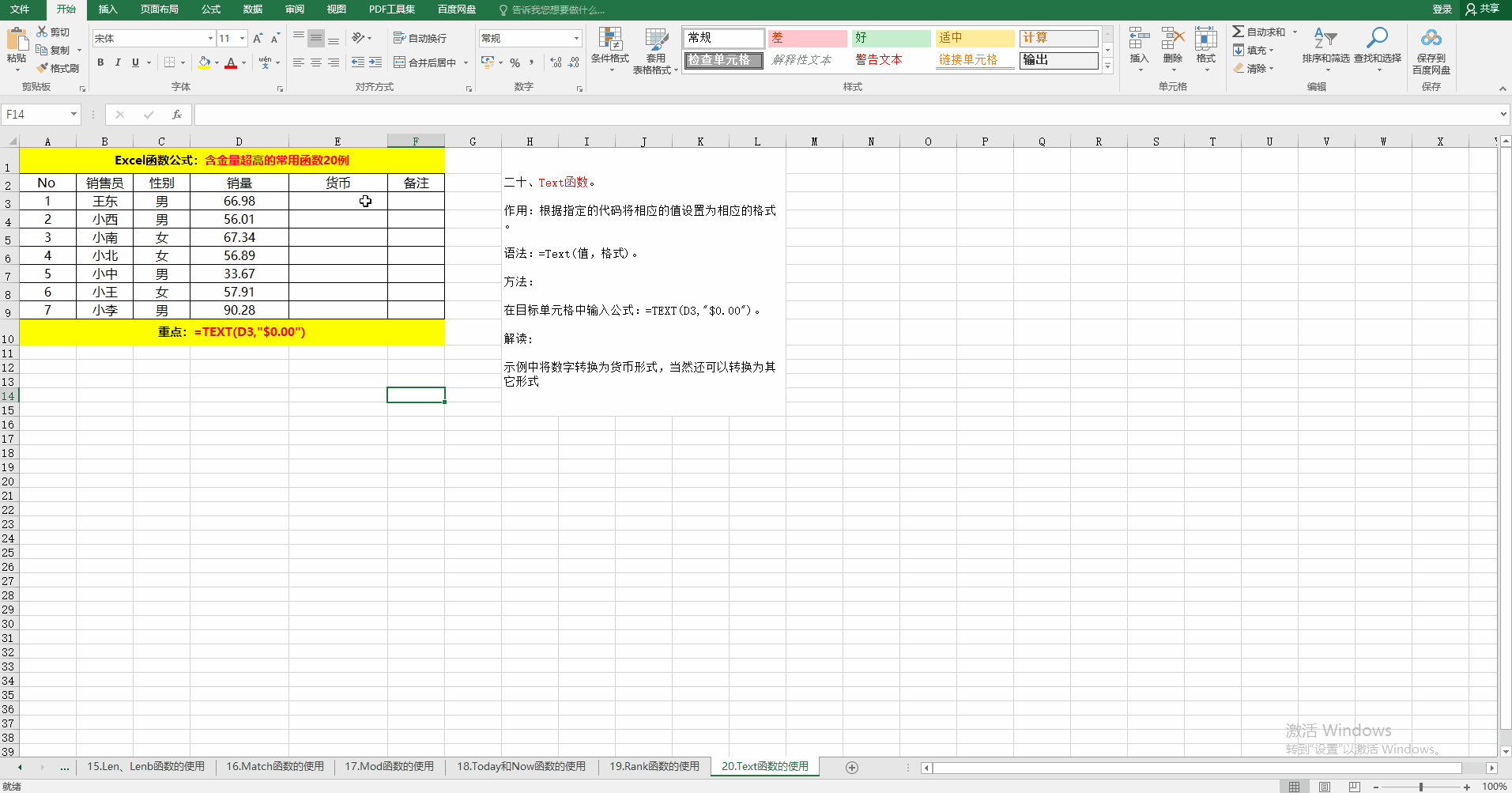附：Excel常用函数公式样例下载地址：https://github.com/13714791953/Excel2016.git

展开全文• 一MATLAB 常用的基本数学函数 abs(x) 纯量的绝对值或向量的长度 angle(z) 复数z 的相角(Phaseangle) sqrt(x)开平方 real(z)复数z 的实部 imag(z)复数z 的虚部 conj(z)复数z 的共轭复数 round(x)四舍入至最近整数 ...
• Excel常用函数汇总及函数的组合用法：包括快捷键和输入的技巧、常用逻辑类、日期时间类、文本类、查找与引用类、数学与三角类、统计类函数，以及综合多函数的用法excel 函数
• excel vba常用函数语法和使用参考，可作为工具查询，希望大家喜欢vba 函数
• 办公行政人员，经常有大量的数据处理，用数据库有点浪费，而且对一般人员来说较难掌握，EXCEL用起来就方便多了，特意整理了一些常用函数，方便使用。
• 组合1VLOOKUP+MATCH常用套路：=VLOOKUP(C12,B2:G9,MATCH(E11,B1:G1,),)套路指南：1、MATCH函数用于返回指定值在某个行或列中的位置。2、VLOOKUP函数是在表格数组的首列查找指定的值，并返回对应行中的其他列的内容。...
• Excel中的统计函数有很多，下面将介绍常见的统计函数的功能，语法，参数以及实例展示。 一、COUNT函数 （1）功能 COUNT函数用于统计参数中包含数字的个数。 （2）格式 =COUNT(value1,[value2],...) 注：...
• 本文档主要讲述excel常用的操作， 常用的公式， 以及常用的函数excel 函数...